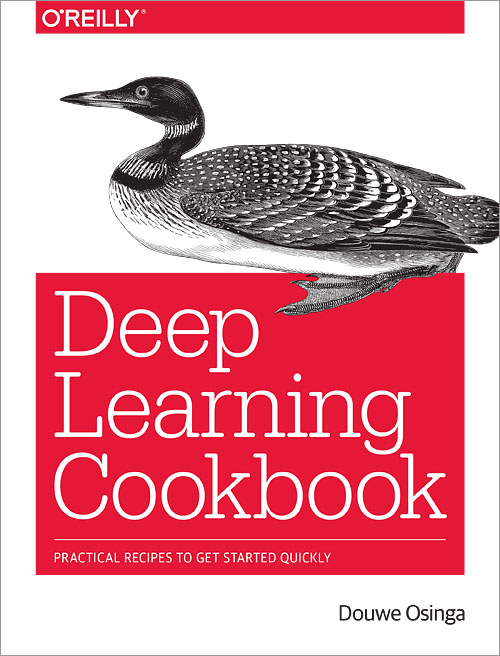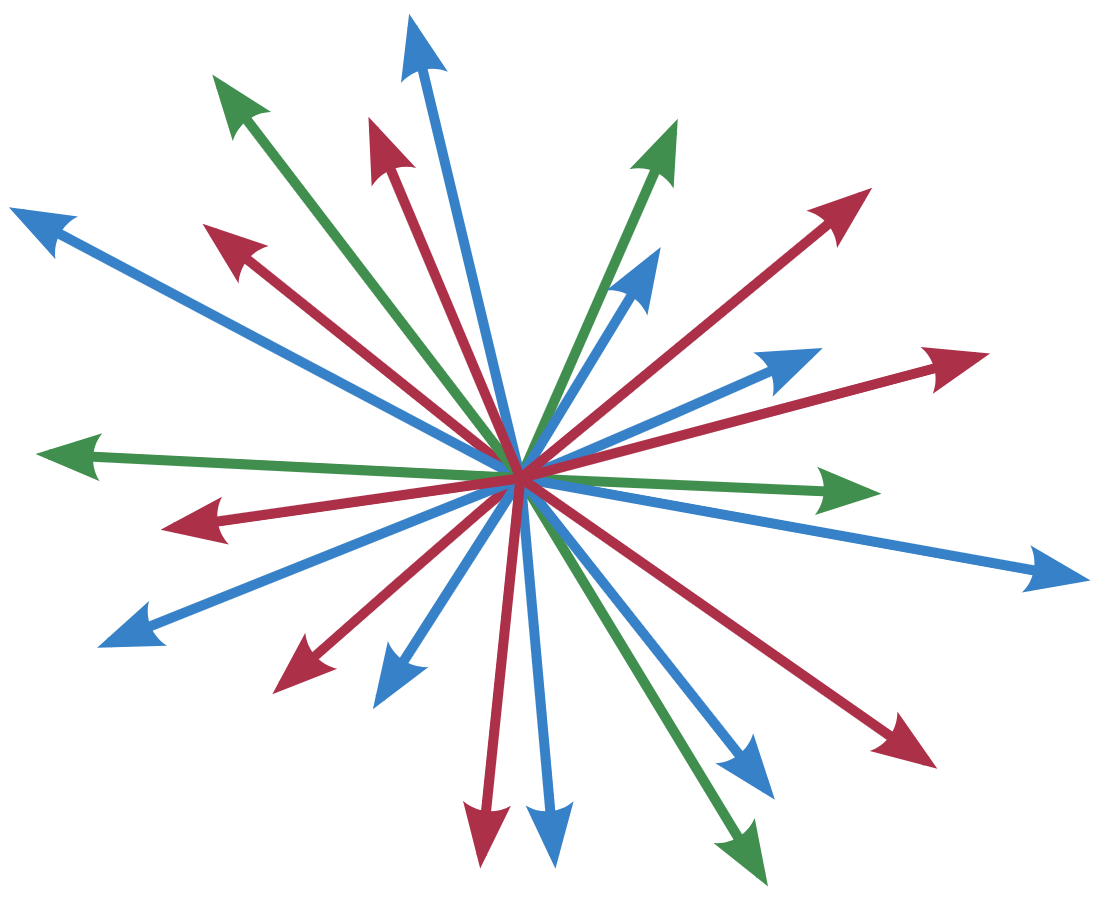# Hacker News Embeddings with PyTorch

Marton Trencseni - Tue 12 March 2019 - Machine Learning

This post is based on Douwe Osinga’s excellent Deep Learning Cookbook, specifically Chapter 4, embeddings. Embedding is a simple thing: given an entity like a Hacker News post or a Hacker News user, we associate an n-dimensional vector with it. We then do a simple thing: if two entities are similar in some way, we assert that the dot product (cosine similarity) should be +1, ie. the vectors should be “aligned”. If two entities are not similar, we assert that the dot product should be -1, ie. they should point in different directions. We then feed the data to a model, and in the training process get the optimizer to find assignments of entities to vectors such that those assertions are satisfied as much as possible. The most famous example of embeddings is Google's word2vec.In the book, embedding is performed on movies. For each movie, the wikipedia page is retrieved, and outgoing links to other wiki pages are collected. Two movies are similar if they both link to the same wiki page, else they are not similar. Keras is used to train the model and the results are reasonably good.

I wanted to implement the same thing in PyTorch, but on a different data set, to keep it interesting. As a regular Hacker News reader, I chose Hacker News. Likes of user are not public, but comments are, so I use that for similarity.

The plan is:

1. Retrieve the top 1,000 HN posts from 2018 by number of comments
2. For each post, retrieve the unique set of users who commented
3. Use these (post, user) pairs for similarity embedding
4. Train with mean squared error (MSE)
5. Use the resulting model to get:
• post similarity: if I like post P, recommend other posts I might like
• user recommendations: I am user U, recommend posts I might like

All the code shown here, with the data files, is up on Github.

## Getting the top 1,000 HN posts

The simplest way to get this is from Google BigQuery, which has a public Hacker News dataset. We can write a SQL query and download the results as a CSV file from the Google Cloud console:

SELECT
id,
descendants,
title
FROM
bigquery-public-data.hacker_news.full
WHERE
timestamp >= "2018-01-01"
AND timestamp <  “2019-01-01”
AND type = "story"
AND score > 1
ORDER BY
2 DESC
LIMIT
1000


The result of this is top_1000_posts.csv.

## Retrieve commenters for top posts

Getting the comments is not practical from BigQuery because the table stores the tree hierarchy (parent_id of the parent comment, but not the post_id), so we’d have to query repeatedly to get all the comments of the post, which is inconvenient. Fortunately there’s an easier way. Algolia has a Hacker News API where we can download one big JSON per post, containing all the comments. The API endpoint for this is:

https://hn.algolia.com/api/v1/items/<post_id>

So we just go through all the posts from the previous step and download each one from Algolia. Getting the set of commenters out of the JSON would be the easiest with json.load(), but this sometimes fails on bad JSON. Instead we use an rxe regexp:

rxe.one('"author":"').one_or_more(rxe.set_except(['"'])).one('"')

The entire code for this download script is on Github. The script caches files, so repeatedly running it doesn’t repeatedly re-download data from Algolia.

The script outputs the (post, user) pairs into post_comments_1000.csv.

## Building the model

PyTorch has a built-in module for Embeddings, which makes building the model simple. It’s essentially a big array, which stores for each entity the assigned high-dimensional vector. In our case, both posts and users are embedded so if there are num_posts posts and num_users users, then num_vectors = num_posts + num_users. So the array has num_vectors row, each row corresponds to that entity’s embedding vector.

PyTorch will then optimize the entries in this array, so that the dot products of the combinations of the vectors are +1 and -1 as specified during training, or as close as possible.The next step is to create a Model which contains the embedding. We implement the forward() function, which just returns the dot product for a minibatch of posts and users, as per the current embedding vectors:

class Model(torch.nn.Module):
def __init__(self, num_vectors, embedding_dim):
super(Model, self).__init__()
self.embedding = torch.nn.Embedding(num_vectors, embedding_dim, max_norm=1.0)
def forward(self, input):
t1 = self.embedding(torch.LongTensor([v for v in input]))
t2 = self.embedding(torch.LongTensor([v for v in input]))
dot_products = torch.bmm(
t1.contiguous().view(len(input), 1, self.embedding.embedding_dim),
t2.contiguous().view(len(input), self.embedding.embedding_dim, 1)
)
return dot_products.contiguous().view(len(input))


Next, we need to write a function to build the minibatches we will use for training. For training, we will pass in existing combinations and “assert” that the dot product should be +1, and some missing combinations with -1:

def build_minibatch(num_positives, num_negatives):
minibatch = []
for _ in range(num_positives):
which = int(len(idx_list) * random())
minibatch.append(idx_list[which] + )
for _ in range(num_negatives):
while True:
post = int(len(posts) * random())
user = min_user_idx + int(len(users) * random())
if post not in idx_user_posts[user]:
break
minibatch.append([post, user] + [-1])
shuffle(minibatch)
return minibatch


Now we can perform the training. We will embed into 50 dimensions, we will use 500 positive and 500 negative combinations per minibatch. We use the Adam optimizer and minimize the mean squared error between our asserted dot products and the actual dot products:

embedding_dim = 50
model = Model(num_vectors, embedding_dim)
loss_function = torch.nn.MSELoss(reduction='mean')
num_epochs = 50
num_positives = 500
num_negatives = 500
for i in range(num_epochs):
for j in range(num_steps_per_epoch):
minibatch = build_minibatch(num_positives, num_negatives)
y = model.forward(minibatch)
target = torch.FloatTensor([v for v in minibatch])
loss = loss_function(y, target)
if i == 0 and j == 0:
print('r: loss = %.3f' % float(loss))
loss.backward(retain_graph=True)
optimizer.step()
print('%s: loss = %.3f' % (i, float(loss)))
# print out some samples to see how good the fit is
minibatch = build_minibatch(5, 5)
y = model.forward(minibatch)
target = torch.FloatTensor([v for v in minibatch])
print('Sample vectors:');
for i in range(5+5):
print('%.3f vs %.3f' % (float(y[i]), float(target[i])))


Output:

r: loss = 1.016
0: loss = 1.009
...
49: loss = 0.633

Sample vectors:
0.319  vs -1.000
0.226  vs -1.000
-0.232 vs -1.000
0.179  vs -1.000
-0.096 vs -1.000
0.395  vs 1.000
0.537  vs 1.000
-0.020 vs 1.000
0.392  vs 1.000
0.141  vs 1.000


We can see that training is able to reduce the MSE by about 40% from the initial random vectors by finding better alignments. That doesn’t sound too good, but it’s good enough for recommendations to work. Let’s write a function to find the closest vectors to a query vector:

def similar_posts_by_title(title):
post_id = title_to_id[title]
pv = get_post_vector(post_id)
dists = []
for other_post in posts:
if other_post == post_id: continue
ov = get_post_vector(other_post)
dist = torch.dot(pv, ov)
dists.append([float(dist), 'https://news.ycombinator.com/item?id=' + other_post, id_to_title[other_post]])
similars = sorted(dists)[-3:]
similars.reverse()
return similars


The entire ipython notebook is on Github. We can use this to find similar posts, it works reasonably well.

Query: 2018 MacBook Pro Review

Posts recommended for: Maro

## Discussion

1. Clearly we could use the text of the posts/comments to gauge similarity, and would get much better results.
2. If the positive/negative ratio of training samples is too different from 1:1, we actually get a significantly lower MSE, but the resulting model is not useful. Why? If we include too many positive pairs where we “assert” +1 for the dot products, the optimizer will just pull all the vectors together to get +1 all the time and reduce MSE. If we include too many negative pairs, it will pull all posts to one vector and all users to the opposing vector, this configuration will mostly satisfy the training criteria and result in a low MSE. (In the book, 1/10 ratio is used, I think it’s accidental that it works in that case.)
3. When emitting the (post, user) pairs, we cut the users, and only keep users who have between 3 and 50 comments. The lower 3 is just to cut out users who don’t connect posts, so won’t be valuable to the embedding similarity training; so this cut makes the training set leaner and meaner. The 50 is to throw out users who comment on a lot posts, and hence pollute the similarity signal during training. Interestingly, without the upper limit of 50, the model doesn’t converge to a useful configuration! This took a lot of playing around to figure out.
4. Notice that when we got recommendations for a user (user-post dot product), the dot product is always significantly lower than in the post-post case (user-user dot products are also lower). The users seem to be more scattered in the high-dimensional space, the posts seem to be in a more tightly packed subspace.
5. Issues/bugs that slowed me down:
• Both posts and users are embedded, so we must remember at which row in the embedding matrix the user vectors start (min_user_idx in the code). Initially I forgot to account for this, both started indexing at 0. Everything ran, but the similarity results were garbage. A nicer solution here would be to use 2 Embedding objects (essentially 2 arrays), so we don’t have to remember the offset.
• I forgot to call optimizer.zero_grad() in the training loop. Everything ran, but the similarity results were garbage. Without the zero_grad() call, the gradients are accumulated, and the optimizer jumps around aimlessly.